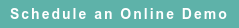# RoyaltyStat Blog

### Posts by Topic

Assets are heterogeneous making cross-companies comparisons difficult. We may get relief knowing that the specific assets composing the “perpetual inventory” dynamic equation of company growth rates can be restricted to property, plant & equipment (PPE); however, different start or acquisition dates (called vintages) and different depreciation rates make PPE also difficult to compare across companies.

In transfer pricing, problems of comparing hetorogeneous assets are exacerbated because regulatory misguidance includes inventory, accounts receivable, and accounts payable in the definition of operating assets. But such inclusions are not warranted.

First, for the “tested party” (controlled entity) importing goods and services from affiliates both inventory and accounts payable are mixed with related-party transactions; thus, they cannot compose the assets base to determine arm’s length profits.

Second, for the tested party exporting goods and services to affiliates inventory and accounts receivable are not free of related party transactions; thus, inventory and accounts receivable cannot configure the assets base to determine arm’s length profits. Also, these short-term assets (inventory, accounts receivable) and liabilities (accounts payable) are not subject to depreciation as PPE, and thus aggregating them with PPE may produce bias.

Including long-term liabilities in operating assets is also misconceived because liabilities are not subject to depreciation and their interest payments are reported after operating profits. Therefore, we can get more traction in theory and empirical measurements when operating assets become restricted to PPE. In this regard, the accounting concept of investment = CAPX is misspecified because investments must include compensation of employees, an important account that is not disclosed to the US Securities & Exchange Commission (SEC).

Arguendo, assume that gross investments ≡ CAPX ≡ G (i.e., excluding compensation of employees), and define PPE (net of accumulated depreciation of tangible assets, excluding amortization of acquired intangibles or intangible impairment charges) ≡ K. Given G and K, we posit a fixed growth rate of gross investment:

(1)    γ ≡ G(t) / K(t – 1)

If, despite our alarm that accounting assets are unreliable, a transfer pricing analyst insists in using assets as the base to determine comparable operating profits, we offer another look at ROA (“return on assets”).

Defining P(t) ≡ EBIT (operating profits after depreciation; again, excluding amortization of acquired intangibles or excluding depletion) and U(t) ≡ random error, and posit:

(2)     P(t) = ρ K(t – 1) + U(t) is the operating profit equation;

(3)     K(t) ≡ G(t) + (1 – δ) K(t – 1)

which is the perpetual inventory equation considering t = 1 to T years of data for each comparable company. See e.g. Klein (1962), pp. 88, 138, 194-195, Kuh (1963), equation 2.3, p. 9, Maddala (1977), pp. 142-146, Usher (1980), p. 6 (equation 6), OECD (2009), Chapter 10 (perpetual inventory method (PIM)).

We can’t estimate the structural (behavioral) equation (2) because K(t) is endogenous. Thus, we consider the equations (1) and (3) to obtain a reduced-form equation:

P(t) = ρ [G(t – 1) +  (1 – δ) K(t – 2)] + U(t)

P(t) = ρ [γ K(t – 2) + (1 – δ) K(t – 2)] + U(t)

(4)    P(t) = π K(t – 2) + U(t)

where π = ρ [γ + (1 – δ)] is a composite coefficient including the depreciation rate.

If (1) is true, equation (4) means that the slope coefficient π = ROA (“return on assets,” where assets are restricted to PPE) of the selected comparable company is the ratio of operating profits after depreciation to PPE lagged two-periods.

In fact, it’s difficult to hold that the assumed depreciation rate (δ) is the same among the selected comparables, and so it’s likely that both γ and the hidden intercept may differ between the selected comparables. Thus, the expected “invisible hand” of gravitation (tendency towards a single ROA among the comparables) may not be achieved.

In sum, the selection of ROA in transfer pricing is clogged with avoidable problems and, perhaps for this same reason, it’s an open grotto for game playing. The Hermes’ thread out of this labyrinth is to produce profit indicators based on more reliable data than accounting measures of assets. We prefer to calculate more reliable profit margins for controlled importers and profit markups for controlled exporters both based on the reduced-form of the price plus profit markup equation.

References

Lawrence Klein, Introduction to Econometrics, Prentice-Hall, 1962.

Edwin Kuh, Capital Stock Growth (A Micro-Econometric Approach), North-Holland, 1963.

Measuring Capital (2nd edition): OECD Manual (2009), which you can read online: https://www.oecd.org/publications/measuring-capital-oecd-manual-2009-9789264068476-en.htm

Dan Usher (editor), The Measurement of Capital (NBER Studies in Income and Wealth Vol. 45), University of Chicago Press, 1980. Edited papers from the conference on the measurement of capital, Toronto, 1976.

Published on Sep 30, 2019 12:32:38 PM

Ednaldo Silva (Ph.D.) is founder and managing director of RoyaltyStat. He helped draft the US transfer pricing regulations and developed the comparable profits method called TNNM by the OECD. He can be contacted at: esilva@royaltystat.com

RoyaltyStat provides premier online databases of royalty rates extracted from unredacted license agreements
and normalized company financials (income statement, balance sheet, cash flow). We provide high-quality data, built-in analytical tools, customer training and attentive technical support.Topics: Net Profit Indicator